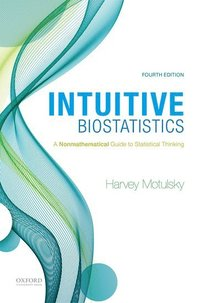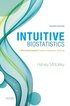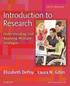Fler böcker inom
Format
Språk
Engelska
Antal sidor
608
Utgivningsdatum
2017-12-21
Upplaga
4
Förlag
OUP USA
Dimensioner
228 x 152 x 25 mm
Vikt
748 g
Antal komponenter
1
ISBN
9780190643560

### Du kanske gillar# Intuitive Biostatistics

A Nonmathematical Guide to Statistical Thinking

1101
Skickas inom 5-8 vardagar.
Fri frakt inom Sverige för privatpersoner.
Finns även som
• Skickas inom 11-20 vardagar (specialorder)
669
.

## Passar bra ihop

1.2. +
3.De som köpt den här boken har ofta också köpt Introduction to Research av Elizabeth Depoy (häftad).

## Kundrecensioner

Har du läst boken? Sätt ditt betyg »

## Recensioner i media

Gilbert S. Omenn, University of Michigan
<"This splendid book meets a major need in public health, medicine, and biomedical research training. It is a user-friendly biostatistics text for non-mathematicians that clearly explains how to make sense of statistical results, avoid common mistakes in data analysis, avoid being confused by statistical nonsense, and how to make research more reproducible. Students may enjoy statistics for the first time!

Louis G. Zachos, University of Mississippi
<"This book applies what I would call <"scientific common sense>" to the confusing world of statistical analysis and interpretation. If you want to really understand what a p-value is, read this book.>

John D. Bonagura, The Ohio State University
<"Intuitive Biostatistics places statistical concepts and practical issues of data analysis within an understandable light. The textbook helps the reader grasp the fundamentals and the pitfalls of data presentation and analysis. It should be on the <"must-read>" list of clinicians, journal reviewers, editors, and other consumers of scientific literature.>

Walter E. Schargel, The University of Texas at Arlington <"I have already recommend the book to many colleagues. A concise, well written, and at times funny book that clearly explains the most important conceptual aspects about statistics, emphasizing proper interpretation of results and common mistakes to avoid.>

## Övrig information

Harvey Motulsky is the CEO and Founder of GraphPad Software, Inc. He wrote the first edition of this text while on the faculty of the Department of Pharmacology at University of California, San Diego.

## Innehållsförteckning

Part A. Introducing Statistics 1. Statistics and Probability are not Intuitive 2. The Complexities of Probability 3. From Sample to Population Part B. Introducing Confidence Intervals 4. Confidence Interval of a Proportion 5. Confidence Interval of Survival Data 6. Confidence Interval of Counted Data (Poisson Distribution) Part C. Continuous Variables 7. Graphing Continuous Data 8. Types of Variables 9. Quantifying Scatter 10. The Gaussian Distribution 11. The Lognormal Distribution and Geometric Mean 12. Confidence Interval of a Mean 13. The Theory of Confidence Intervals 14. Error Bars Part D. P Values and Statistical Significance 15. Introducing P Values 16. Statistical Significance and Hypothesis Testing 17. Comparing Groups with Confidence Intervals and P Values 18. Interpreting a Result That Is Statistically Significant 19. Interpreting a Result That Is Not Statistically Significant 20. Statistical Power 21. Testing For Equivalence or Noninferiority Part E. Challenges in Statistics 22. Multiple Comparisons Concepts 23. The Ubiquity of Multiple Comparisons 24. Normality Tests 25. Outliers 26. Choosing a Sample Size Part F. Statistical Tests 27. Comparing Proportions 28. Case-Control Studies 29. Comparing Survival Curves 30. Comparing Two Means: Unpaired t Test 31. Comparing Two Paired Groups 32. Correlation Part G. Fitting Models to Data 33. Simple Linear Regression 34. Introducing Models 35. Comparing Models 36. Nonlinear Regression 37. Multiple Regression 38. Logistic and Proportional Hazards Regression Part H. The Rest of Statistics 39. Analysis of Variance 40. Multiple Comparison Tests after ANOVA 41. Nonparametric Methods 42. Sensitivity, Specificity, and Receiver-Operating Characteristic Curves 43. Meta-Analysis Part I. Putting It All Together 44. The Key Concepts of Statistics 45. Statistical Traps to Avoid 46. Capstone Example 47. Statistics and Reproducibility 48. Checklists for Reporting Statistical Methods and Results Part J. Appendices